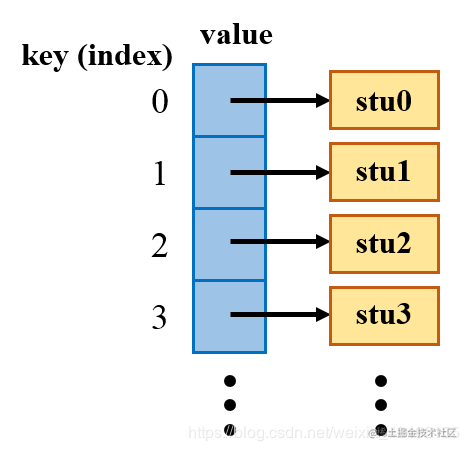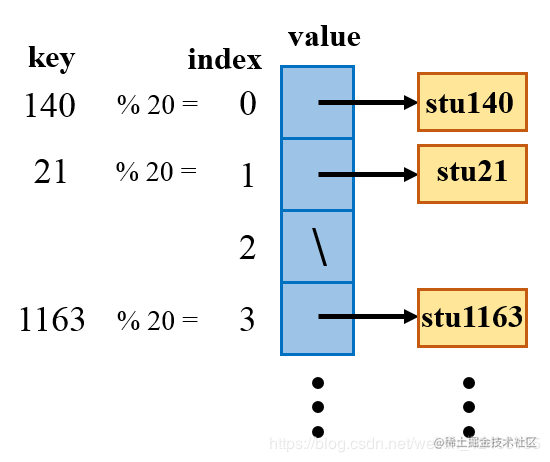# HashMap 实现原理及源码分析

HashMap是JDK中非常重要的容器，采用 数组 + 链表 的方式实现，理想情况下能支持 O(1) 时间复杂度的增删改查操作。本文将由浅入深地讲解哈希表的实现原理，并对HashMap的部分源码进行分析。

## 1. 从数组说起

``````public class Student {
public int studentID;
public String name;
public Student(int studentID, String name) {
this.studentID = studentID;
this.name = name;
}
} 复制代码``````

``````Student[] students = new Student;
Student stu0 = new Student(0, "stu0");
Student stu19 = new Student(19, "stu19");

students[stu0.studentID] = stu0;
students[stu19.studentID] = stu19; 复制代码``````## 2. 哈希函数和哈希碰撞

``````Student stu21 = new Student(21, "stu21");
Student stu140 = new Student(140, "stu140");
Student stu1163 = new Student(1163, "stu1163");

students[stu21.studentID % 20] = stu21;
students[stu140.studentID % 20] = stu140;
students[stu1163.studentID % 20] = stu1163; 复制代码``````## 3. HashMap 中的常量

HashMap 中的一些比较重要的常量如下。

``````static final int DEFAULT_INITIAL_CAPACITY = 1 << 4;
static final float DEFAULT_LOAD_FACTOR = 0.75f;
static final int TREEIFY_THRESHOLD = 8; 复制代码``````

TREEIFY_THRESHOLD 是树形化阈值，当某个 slot 悬挂的结点数量大于树形化阈值的时候，就把链表转化为一棵红黑树。为什么树形化阈值取 8 呢？按照官方的说法，如果 key 的哈希值是随机的，在装载因子为 0.75 时，每个 slot 中节点出现的频率服从参数为 0.5 的泊松分布，所以链表的长度（size）为 k 的概率为## 4. 静态内部类 Node

node 就是结点的意思，本文中所说的 “结点”，指的就是一个 Node 对象。Node<K, V> 实现了 Entry<K, V> 接口。

``static class Node<K,V> implements Map.Entry<K,V> 复制代码``

Node<V, U> 中有 4 个成员变量。可以看出 Node 的主要功能是把 key 和与之对应的 value 封装到一个结点中，该结点的 next 字段指向下一个结点，从而实现单向链表。hash 由 key 的哈希值得来，下文会介绍。

``````final int hash;
final K key;
V value;
Node<K,V> next; 复制代码``````

## 5. HashMap 构造函数和扩容

``````transient Node<K,V>[] table;
transient int size;
int threshold;

``````public HashMap(int initialCapacity, float loadFactor) {
// 省略了一些判断极端输入的代码
this.threshold = tableSizeFor(initialCapacity);
}
public HashMap() {
} 复制代码``````

tableSizeFor() 这个方法返回大于等于 initialCapaticy 的最小的 2 的幂。比如输入 16 就返回 16，输入 17 就返回 32。以下是该方法的实现。

``````static final int tableSizeFor(int cap) {
int n = -1 >>> Integer.numberOfLeadingZeros(cap - 1);
return (n < 0) ? 1 : (n >= MAXIMUM_CAPACITY) ? MAXIMUM_CAPACITY : n + 1;
} 复制代码``````

``````final Node<K,V>[] resize() {
Node<K,V>[] oldTab = table;
int oldCap = (oldTab == null) ? 0 : oldTab.length;
int oldThr = threshold;
int newCap, newThr = 0;
if (oldCap > 0) {
if (oldCap >= MAXIMUM_CAPACITY) {
threshold = Integer.MAX_VALUE;
return oldTab;
}
else if ((newCap = oldCap << 1) < MAXIMUM_CAPACITY &&
oldCap >= DEFAULT_INITIAL_CAPACITY)
newThr = oldThr << 1; // double threshold
}
else if (oldThr > 0) // initial capacity was placed in threshold
newCap = oldThr;
else {               // zero initial threshold signifies using defaults
newCap = DEFAULT_INITIAL_CAPACITY;
}
if (newThr == 0) {
float ft = (float)newCap * loadFactor;
newThr = (newCap < MAXIMUM_CAPACITY && ft < (float)MAXIMUM_CAPACITY ?
(int)ft : Integer.MAX_VALUE);
}
threshold = newThr;
@SuppressWarnings({"rawtypes","unchecked"})
Node<K,V>[] newTab = (Node<K,V>[])new Node[newCap];
table = newTab;
if (oldTab != null) {
for (int j = 0; j < oldCap; ++j) {
Node<K,V> e;
if ((e = oldTab[j]) != null) {
oldTab[j] = null;
if (e.next == null)
newTab[e.hash & (newCap - 1)] = e;
else if (e instanceof TreeNode)
((TreeNode<K,V>)e).split(this, newTab, j, oldCap);
else { // preserve order
Node<K,V> loHead = null, loTail = null;
Node<K,V> hiHead = null, hiTail = null;
Node<K,V> next;
do {
next = e.next;
if ((e.hash & oldCap) == 0) {
if (loTail == null)
else
loTail.next = e;
loTail = e;
}
else {
if (hiTail == null)
else
hiTail.next = e;
hiTail = e;
}
} while ((e = next) != null);
if (loTail != null) {
loTail.next = null;
}
if (hiTail != null) {
hiTail.next = null;
}
}
}
}
}
return newTab;
} 复制代码``````

## 6. put() 和 get() 方法

``````Student stu21 = new Student(21, "stu21");
HashMap<Integer, Student> map = new HashMap<>();
map.put(stu21.studentID, stu21); 复制代码``````

stu21.studentID 是 int 类型，在执行 put() 方法之前，需要进行装箱，把它转换为 Integer 类型，这一过程由编译器自动完成。

``````public V put(K key, V value) {
return putVal(hash(key), key, value, false, true);
} 复制代码``````

put() 方法中调用了 putVal() 方法。如下所示，其中的 hash() 方法是一个静态方法。返回的是 key 的哈希值无符号右移 16 位，然后跟自身异或的结果。其目的是为了利用哈希值前 16 位的信息。

``````static final int hash(Object key) {
int h;
return (key == null) ? 0 : (h = key.hashCode()) ^ (h >>> 16);
} 复制代码``````

``````final V putVal(int hash, K key, V value, boolean onlyIfAbsent,
boolean evict) {
Node<K,V>[] tab; Node<K,V> p; int n, i;
if ((tab = table) == null || (n = tab.length) == 0)
n = (tab = resize()).length;
if ((p = tab[i = (n - 1) & hash]) == null)
tab[i] = newNode(hash, key, value, null);
else {
Node<K,V> e; K k;
if (p.hash == hash &&
((k = p.key) == key || (key != null && key.equals(k))))
e = p;
else if (p instanceof TreeNode)
e = ((TreeNode<K,V>)p).putTreeVal(this, tab, hash, key, value);
else {
for (int binCount = 0; ; ++binCount) {
if ((e = p.next) == null) {
p.next = newNode(hash, key, value, null);
if (binCount >= TREEIFY_THRESHOLD - 1) // -1 for 1st
treeifyBin(tab, hash);
break;
}
if (e.hash == hash &&
((k = e.key) == key || (key != null && key.equals(k))))
break;
p = e;
}
}
if (e != null) { // existing mapping for key
V oldValue = e.value;
if (!onlyIfAbsent || oldValue == null)
e.value = value;
afterNodeAccess(e);
return oldValue;
}
}
++modCount;
if (++size > threshold)
resize();
afterNodeInsertion(evict);
return null;
} 复制代码``````

get() 方法相对来讲就比较简单了，不做过多解释。

``````public V get(Object key) {
Node<K,V> e;
return (e = getNode(hash(key), key)) == null ? null : e.value;
} 复制代码``````

``````final Node<K,V> getNode(int hash, Object key) {
Node<K,V>[] tab; Node<K,V> first, e; int n; K k;
if ((tab = table) != null && (n = tab.length) > 0 &&
(first = tab[(n - 1) & hash]) != null) {
if (first.hash == hash && // always check first node
((k = first.key) == key || (key != null && key.equals(k))))
return first;
if ((e = first.next) != null) {
if (first instanceof TreeNode)
return ((TreeNode<K,V>)first).getTreeNode(hash, key);
do {
if (e.hash == hash &&
((k = e.key) == key || (key != null && key.equals(k))))
return e;
} while ((e = e.next) != null);
}
}
return null;
} 复制代码``````

## 推荐阅读：